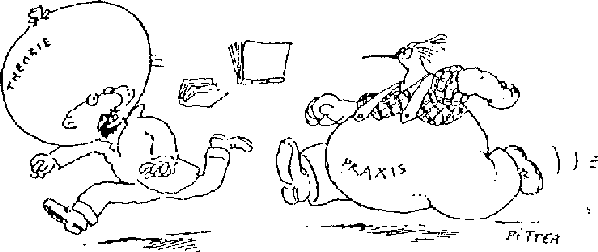## Density functional theory & practice

Spezialvorlesung, Universität Stuttgart, WS 2003/04, Do 14:00-15:30, V 57.05
E. Koch, MPI-FKF

In 1929, P.A.M. Dirac noted that with the advent of quantum mechanics "The fundamental laws necessary for the mathematical treatment of large parts of physics and the whole of chemistry are thus fully known, and the difficulty lies only in the fact that application of these laws leads to equations that are too complex to be solved." Mainly because the many-body wavefunction for N electrons is a function of 3 N variables: Ψ(r1, r2, …,rN). Only in the sixties it was recognized that a system of interacting electrons is completely characterized by the electron density n(r) in the ground state. With the density as a basic variable one only has to find a function of 3 variables, independently of the number of electrons in the system. Unfortunately the equations for determining the density are not known so that one has to work with approximate equations. The success of density functional theory is based on the fact that very good approximations have been found — and on the availability of computers of ever increasing speed. This allows practical calculations of numerous physical properties for vast classes of molecules and solids. These achievements were recognized with the 1998 Nobel Prize in Chemistry to Walter Kohn.

In the lecture we will explain the basic concepts of density functional theory and discuss practical aspects. The main goal is to give a feeling of what can be done with these methods (which are by now implemented in standard codes that are sometimes even freely available) and where such calculations can be trusted. As practical applications we will discuss how to calculate and interpret band structures, how to do Car-Parrinello molecular dynamics, and we will address approaches for calculating excited states.### Contents of Lectures

1. Introduction & perspective: slides
2. Theorems: slides
• density matrices; exchange-correlation hole
• coupling-constant integration
• Hohenberg-Kohn: The density as the basic variable
• Kohn-Sham: mapping to single-electron problem
3. Practice: slides
4. Applications:
• ground state: energy, structure, elastic constants
• forces: molecular dynamics
• excited states?
• parameters for correlated systems
• No.3: Ground State of the Electron Gas by a Stochastic Method (D.M. Ceperley and B.J. Alder)
• No.5: Unified Approach for Molecular Dynamics and Density Functional Theory (R. Car and M. Parrinello)

Some DFT conferences:

Erik Koch